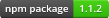# gql

Query language for interpreting genome SNPs

## Usage no npm install needed!

``````<script type="module">
import gql from 'https://cdn.skypack.dev/gql';
</script>``````## Information

 Package gql Description Query language for interpreting genome SNPs Node Version >= 0.4

## Compatibility

This query language is for use with the genomejs JSON format. See the dna2json repository for more information.

Just a reminder: deletions are represented as `-`!

## Usage

This example will create a query that determines if a person is sickle cell effected.

``````var gql = require('gql');
var dna = require('./genome.json');

var query = gql.or([
gql.exact('rs334', 'TT'),
gql.exact('i3003137', 'AA')
]);

var isMatch = query(dna);
console.log(isMatch); // true or false
``````

## API

All of these APIs return a boolean-returning function that acts as the truth test. These functions take in one argument, a DNA-JSON object. You can nest as deep as you want, or be as basic as you want.

For example, checking if a person is immune to norovirus is pretty simple:

``````var dna = require('./my-dna.json');

var query = gql.exact('rs601338', 'AA');
var isImmune = query(dna);
``````

### Conditions

#### exact(id, genotype)

Evaluates to true if this was the only allele observed.

``````q.exact('rs2032651', 'D'); // will only match genotype D
q.exact('rs2032651', 'AT'); // will only match genotype AT
``````

#### has(id, genotype)

Evaluates to true if the allele was observed at all.

``````q.has('rs2032651', 'A'); // will match GA, GAT, AB, etc.
``````

#### exists(id)

Evaluates to true if any allele has been observed.

``````q.exists('rs2032651');
``````

#### doesntExist(id)

Evaluates to true if no allele has been observed.

``````q.doesntExist('rs2032651');
``````

#### not(condition)

Inverts the result of another condition.

``````// evaluates to true if rs2032651 != AA
var query = q.not(q.exact('rs2032651', 'AA'));

query({
rs2032651: {
genotype: 'TT'
}
}); // true

query({
rs2032651: {
genotype: 'AA'
}
}); // false
``````

#### only(id, allele)

Evaluates to true if only the allele was observed at the specified position.

``````q.only('rs2032651', 'A'); // will match A, AA, AAA, etc.
``````

### Aggregate Conditions

#### or(conditions...)

Evaluates to true if any of the given condition functions evaluate to true.

``````q.or([
q.has('rs2032651', 'A'),
q.doesntExist('rs2032651')
]);
``````

#### and(conditions...)

Evaluates to true if all of the given condition functions evaluate to true.

``````q.and([
q.exists('rs2032652'),
q.has('rs2032651', 'A')
]);
``````

#### atLeast(number, conditions...)

Evaluates to true if the number of given condition functions evaluating to true is equal to or greater than the given number.

``````q.atLeast(2, [
q.exists('rs2032652'),
q.has('rs2032651', 'A'),
q.exact('rs2032653', 'AT'),
q.exact('rs2032654', 'GG')
]);
``````

#### none(conditions...)

Evaluates to true if all of the given condition functions evaluate to false.

``````q.none([
q.has('rs2032651', 'A'),
q.doesntExist('rs2032651')
]);
``````